# Merge two Trees using Node Sum | Recursive solution (Set 1)

In this article, we are going to see how to find merge of two given trees using node sum. Here we have used a recursive approach to solve this?
Submitted by Radib Kar, on September 10, 2020

Here, we are going to see how to how to merge two trees using node sum?

In the below example, the two given trees are shown below: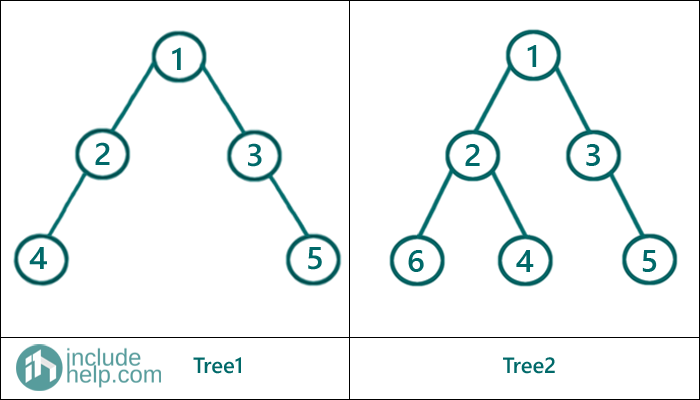After merging the above will return the below tree: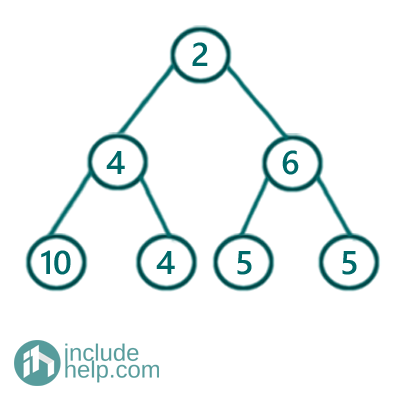Figure 1: Merged Tree (Output)

Here, we have solved using a recursive approach, below is the algorithm:

```Function MergeTree(root1 , root2)
1)  Base cases:
If both roots are NULL
return NULL;
If one of the roots is NULL
Return the Non-NULL node
2)  Creating a new node using the root's sum
Left child of the newly created node = MergeTree(left child of root1, left child of root2)
Right child of the newly created node = MergeTree(right child of root1, right child of root2)
Return newly created node
```

Dry run:

Both roots are not NULL, hence new root is created like below and then we recur for the subtrees,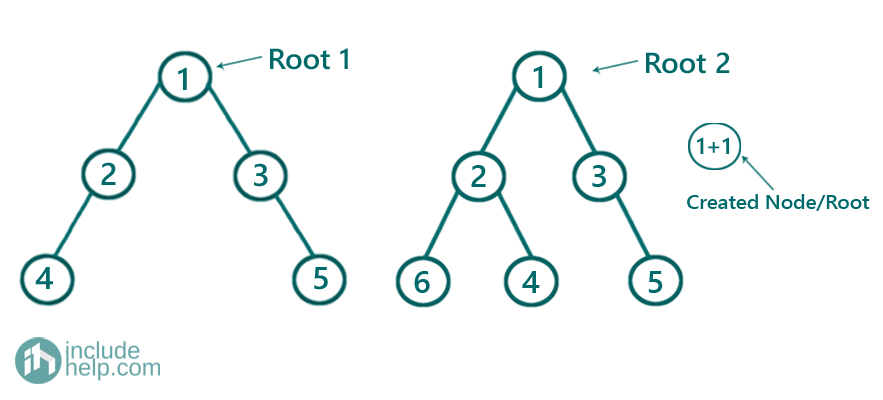Now we go for the left subtrees of (1,1) and again both roots are not NULL.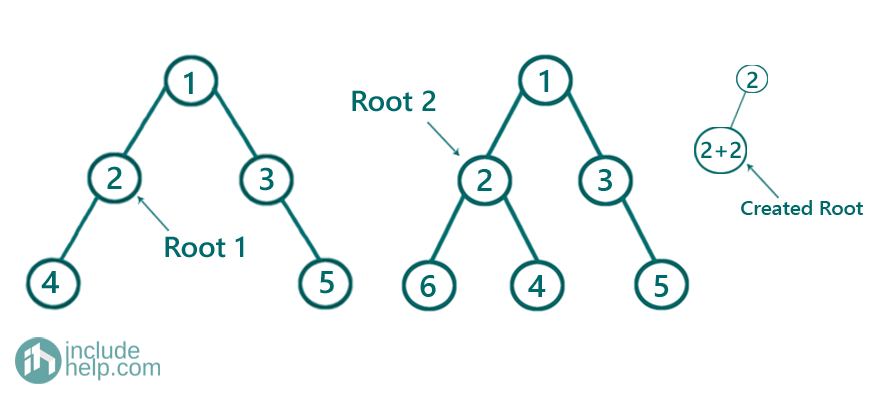Then, we go for left subtrees of (2,2) and again both roots are not NULL.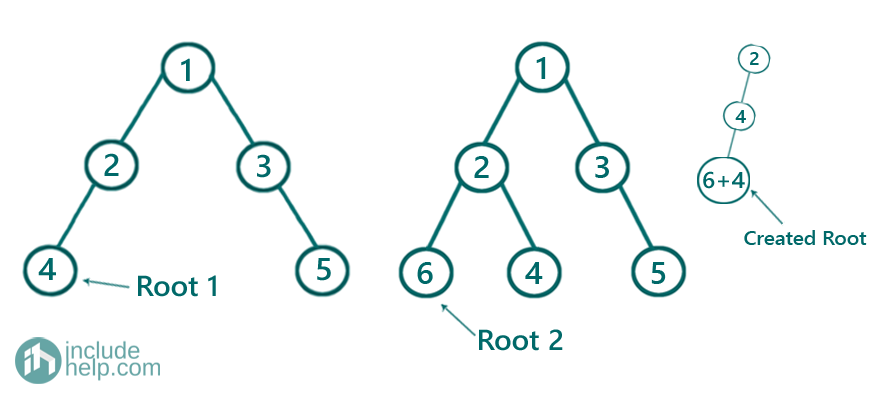While going for the right subtrees of (2, 2) one is NULL and another is not NULL. So we place the Non-NULL node

Below are the further recursive steps: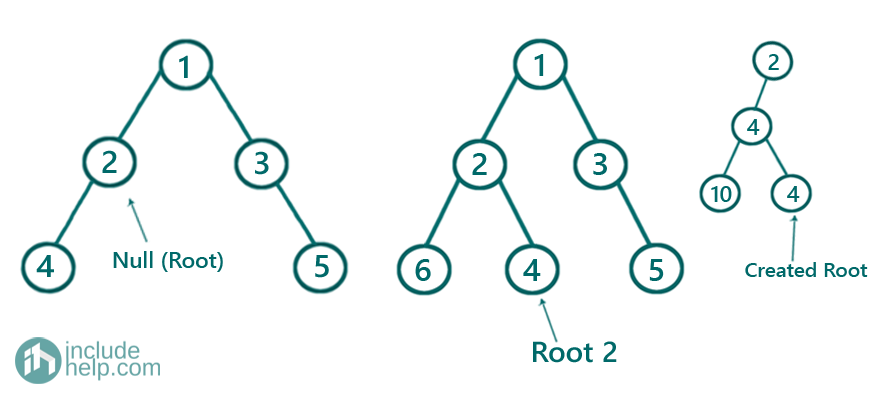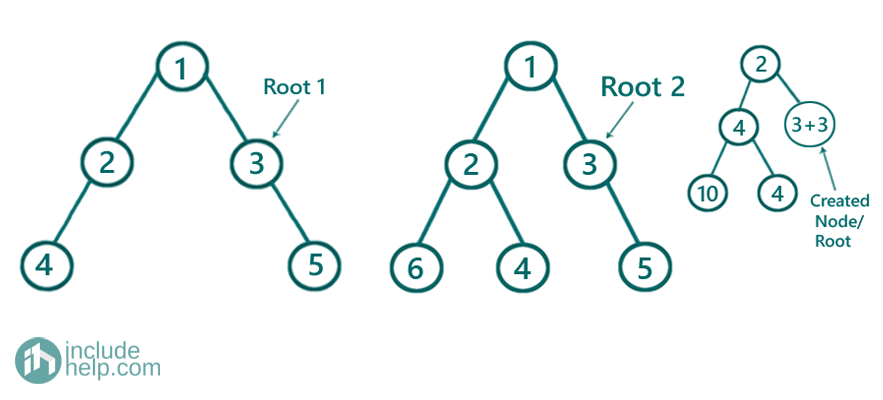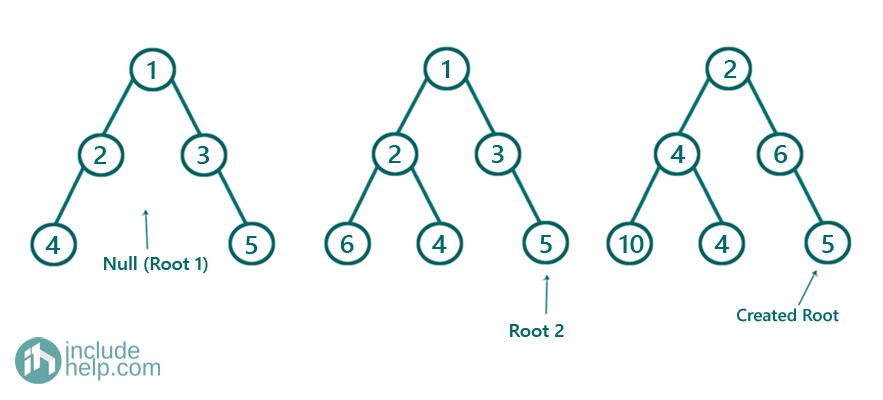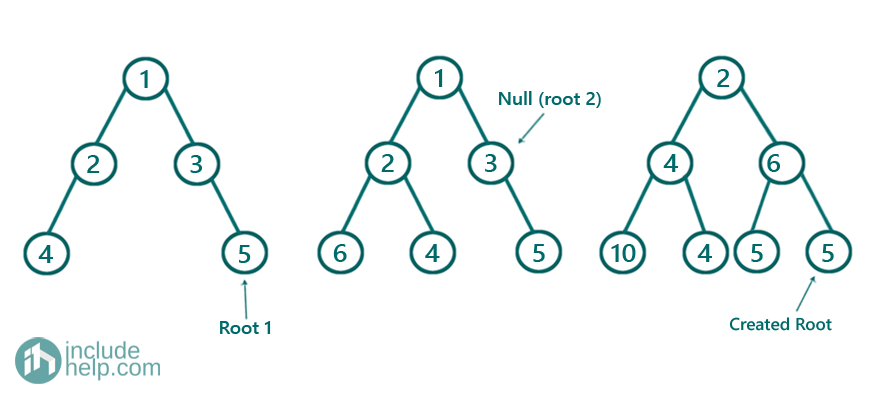Finally, we have: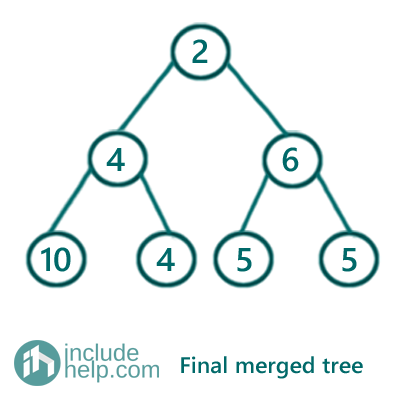C++ Implementation:

```#include <bits/stdc++.h>
using namespace std;

// tree node is defined
class TreeNode {
public:
int val;
TreeNode* left;
TreeNode* right;
TreeNode(int data)
{
val = data;
left = NULL;
right = NULL;
}
};

TreeNode* mergeTrees(TreeNode* t1, TreeNode* t2)
{
if (!t1 && !t2)
return NULL;
if (!t1 || !t2)
return (!t1) ? t2 : t1;

TreeNode* root = new TreeNode(t1->val + t2->val);
root->left = mergeTrees(t1->left, t2->left);
root->right = mergeTrees(t1->right, t2->right);
return root;
}

//inorder traversal
void inorder(TreeNode* root)
{
if (!root)
return;
inorder(root->left);
cout << root->val << " ";
inorder(root->right);
}

int main()
{

//Trees same as example

//Tree 1
TreeNode* root1 = new TreeNode(1);
root1->left = new TreeNode(2);
root1->right = new TreeNode(3);
root1->left->left = new TreeNode(4);
root1->right->right = new TreeNode(5);

//Tree2
TreeNode* root2 = new TreeNode(1);
root2->left = new TreeNode(2);
root2->right = new TreeNode(3);
root2->left->left = new TreeNode(6);
root2->left->right = new TreeNode(4);
root2->right->left = new TreeNode(5);

//resultant tree
TreeNode* root = mergeTrees(root1, root2);

cout << "Inorder traversal of the resulting tree is:\n";

inorder(root);

cout << "\n";

return 0;
}
```

Output:

```Inorder traversal of the resulting tree is:
10 4 4 2 5 6 5
```

What's New

Top Interview Coding Problems/Challenges!

Languages: » C » C++ » C++ STL » Java » Data Structure » C#.Net » Android » Kotlin » SQL
Web Technologies: » PHP » Python » JavaScript » CSS » Ajax » Node.js » Web programming/HTML
Solved programs: » C » C++ » DS » Java » C#
Aptitude que. & ans.: » C » C++ » Java » DBMS
Interview que. & ans.: » C » Embedded C » Java » SEO » HR
CS Subjects: » CS Basics » O.S. » Networks » DBMS » Embedded Systems » Cloud Computing
» Machine learning » CS Organizations » Linux » DOS
More: » Articles » Puzzles » News/Updates